# MPI topic: Functional parallelism

##### Experimental html version of Parallel Programming in MPI, OpenMP, and PETSc by Victor Eijkhout. download the textbook at https:/theartofhpc.com/pcse

$\newcommand\inv{^{-1}}\newcommand\invt{^{-t}} \newcommand\bbP{\mathbb{P}} \newcommand\bbR{\mathbb{R}} \newcommand\defined{ \mathrel{\lower 5pt \hbox{{\equiv\atop\mathrm{D}}}}}$ 2.1 : The SPMD model
2.2 : Starting and running MPI processes
2.2.2 : Initialization / finalization
2.2.2.1 : Aborting an MPI run
2.2.2.2 : Testing the initialized/finalized status
2.2.2.3 : Information about the run
2.2.2.4 : Commandline arguments
2.3 : Processor identification
2.3.1 : Processor name
2.3.2 : Communicators
2.3.3 : Process and communicator properties: rank and size
2.4 : Functional parallelism
2.5 : Distributed computing and distributed data
2.6 : Review questions

# 2 MPI topic: Functional parallelism

## 2.1 The SPMD model

crumb trail: > mpi-functional > The SPMD model

MPI programs conform largely to the SPMD model, where each processor runs the same executable. This running executable we call a process  .

When MPI was first written, 20 years ago, it was clear what a processor was: it was what was in a computer on someone's desk, or in a rack. If this computer was part of a networked cluster, you called it a node  . So if you ran an MPI program, each node would have one MPI process;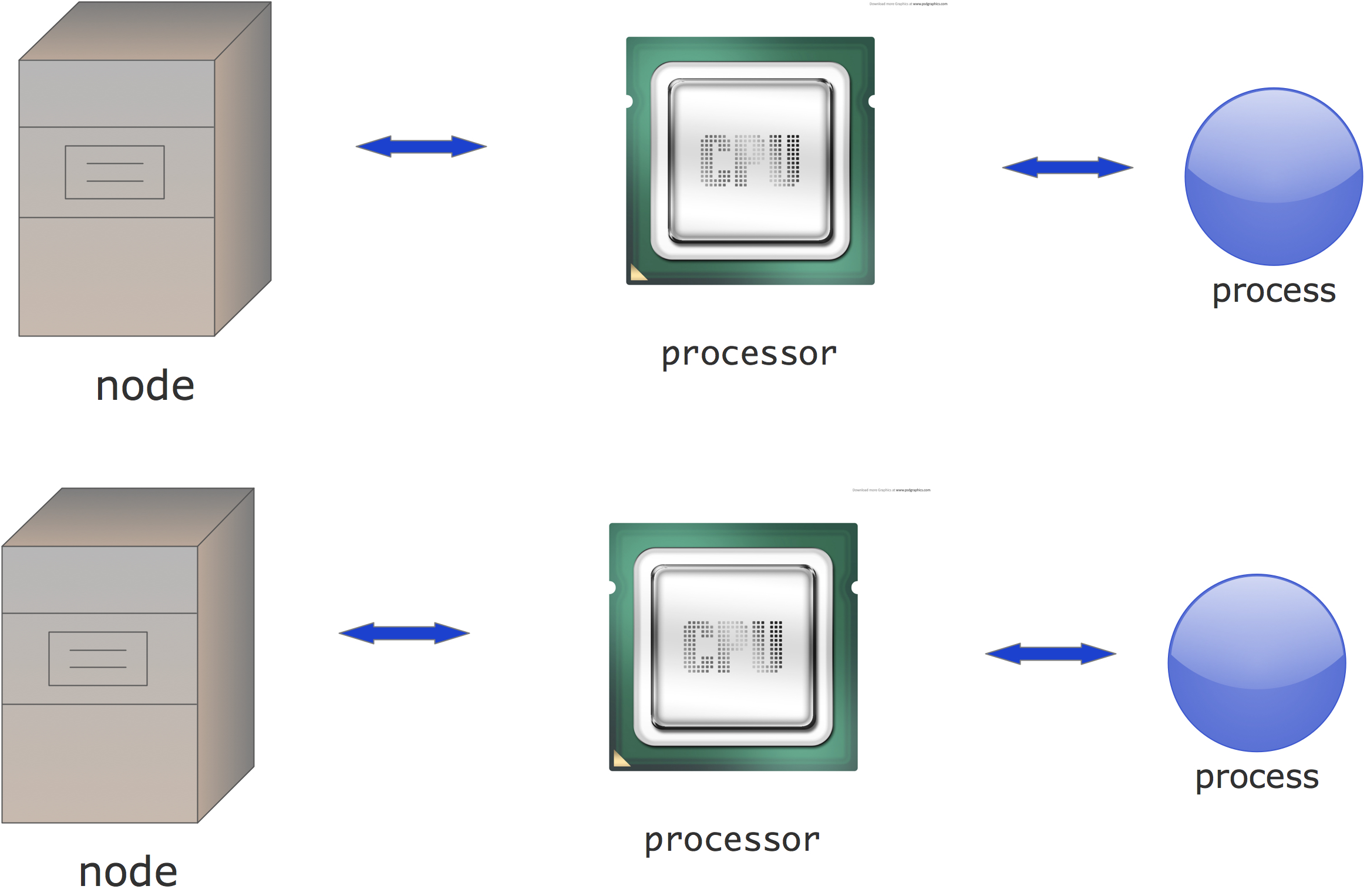FIGURE 2.1: Cluster structure as of the mid 1990s

figure  2.1  . You could of course run more than one process, using the time slicing of the OS  , but that would give you no extra performance.

These days the situation is more complicated. You can still talk about a node in a cluster, but now a node can contain more than one processor chip (sometimes called a socket  ), and each processor chip probably has multiple cores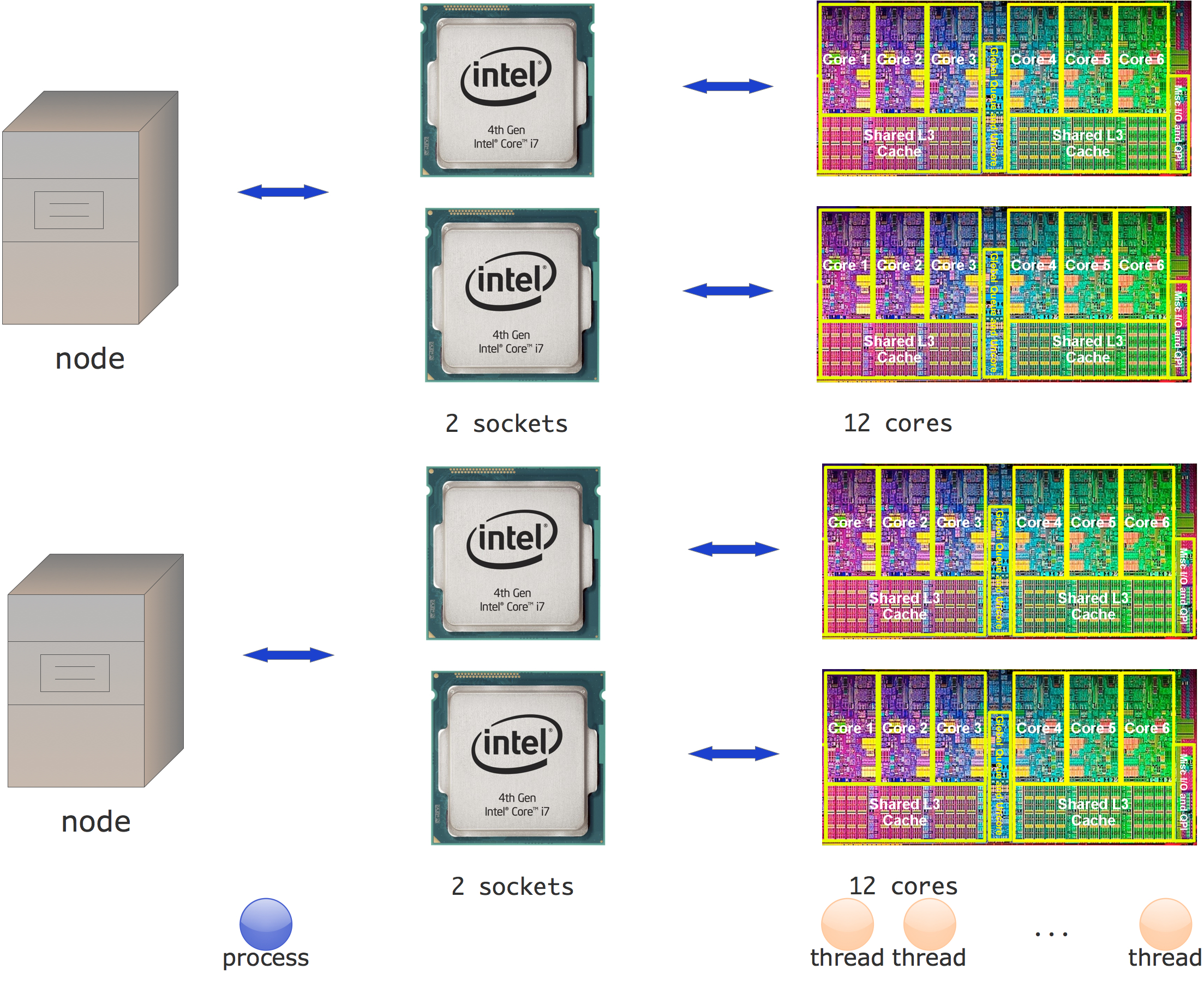FIGURE 2.2: Hybrid cluster structure

Figure  2.2 shows how you could explore this using a mix of MPI between the nodes, and a shared memory programming system on the nodes.

However, since each core can act like an independent processor, you can also have multiple MPI processes per node. To MPI, the cores look like the old completely separate processors. This is the pure MPI' model of figure  2.3  , which we will use in most of this part of the book. (Hybrid computing will be discussed in chapter  Hybrid computing  .)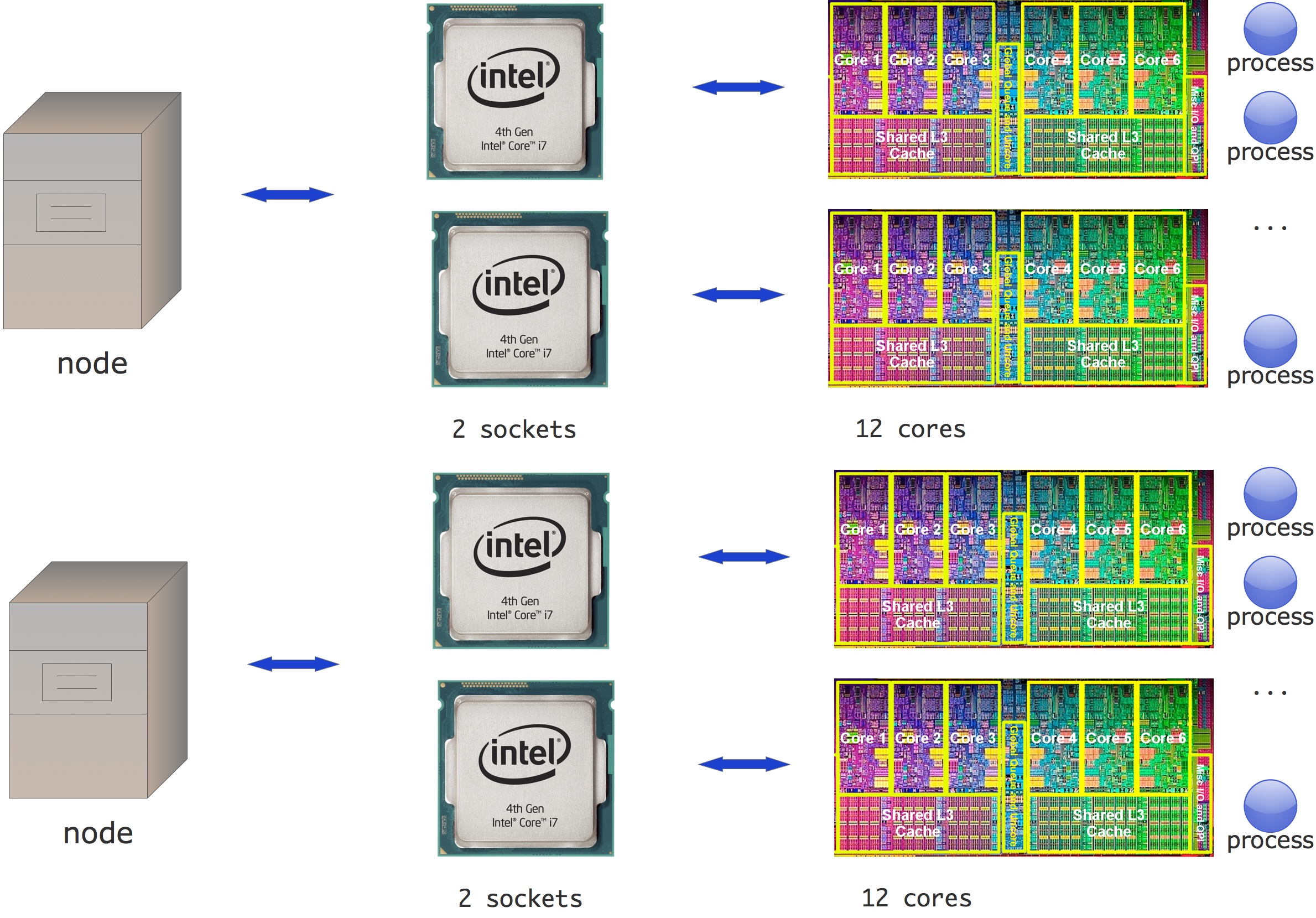FIGURE 2.3: MPI-only cluster structure

This is somewhat confusing: the old processors needed MPI programming, because they were physically separated. The cores on a modern processor, on the other hand, share the same memory, and even some caches. In its basic mode MPI seems to ignore all of this: each core receives an MPI process and the programmer writes the same send/receive call no matter where the other process is located. In fact, you can't immediately see whether two cores are on the same node or different nodes. Of course, on the implementation level MPI uses a different communication mechanism depending on whether cores are on the same socket or on different nodes, so you don't have to worry about lack of efficiency.

Remark In some rare cases you may want to run in an MPMD mode, rather than SPMD  . This can be achieved either on the OS level (see section  15.9.4  ), using options of the mpiexec mechanism, or you can use MPI's built-in process management; chapter  MPI topic: Process management  . Like I said, this concerns only rare cases.
End of remark

## 2.2 Starting and running MPI processes

crumb trail: > mpi-functional > Starting and running MPI processes

The SPMD model may be initially confusing. Even though there is only a single source, compiled into a single executable, the parallel run comprises a number of independently started MPI processes (see section  1.3 for the mechanism).

The following exercises are designed to give you an intuition for this one-source-many-processes setup. In the first exercise you will see that the mechanism for starting MPI programs starts up independent copies. There is nothing in the source that says and now you become parallel'.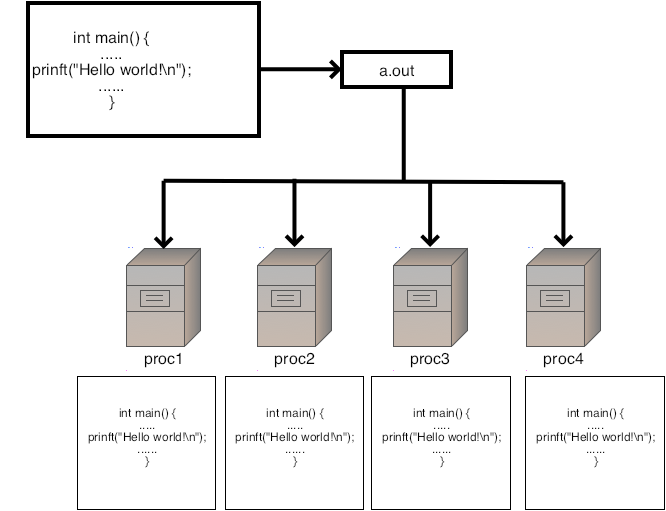FIGURE 2.4: Running a hello world program in parallel

The following exercise demonstrates this point.

Exercise Write a hello world' program, without any MPI in it, and run it in parallel with mpiexec or your local equivalent. Explain the output.

(There is a skeleton code hello in the repository)
End of exercise

This exercise is illustrated in figure  2.4  .

crumb trail: > mpi-functional > Starting and running MPI processes > Headers

If you use MPI commands in a program file, be sure to include the proper header file, mpi.h for C/C++.

#include "mpi.h" // for C

The internals of these files can be different between MPI installations, so you can not compile one file against one mpi.h file and another file, even with the same compiler on the same machine, against a different MPI.

Fortran note For MPI use from Fortran, use an MPI module.

use mpi     ! pre 3.0
use mpi_f08 ! 3.0 standard


New language developments, such as large counts; section  6.4.2 will only be included in the mpi_f08 module, not in the earlier mechanisms.

The header file mpif.h is deprecated as of MPI-4.1: it may be supported by installations, but doing so is strongly discouraged. End of Fortran note

Python note It's easiest to

from mpi4py import MPI


MPL note To compile MPL programs, add a line

#include <mpl/mpl.hpp>

to your file. End of MPL note

### 2.2.2 Initialization / finalization

Every (useful) MPI program has to start with MPI initialization through a call to MPI_Init  , and have MPI_Finalize to finish the use of MPI in your program. The init call is different between the various languages.

In C, you can pass argc and argv  , the arguments of a C language main program:

int main(int argc,char **argv) {
....
return 0;
}

(It is allowed to pass NULL for these arguments.)

Fortran (before 2008) lacks this commandline argument handling, so MPI_Init lacks those arguments.

After MPI_Finalize no MPI routines (with a few exceptions such as MPI_Finalized  ) can be called. In particular, it is not allowed to call MPI_Init again. If you want to do that, use the sessions model ; section  8.3  .

Python note In many cases, no initialize and finalize calls are needed: the statement

## mpi.py
from mpi4py import MPI

performs the initialization. Likewise, the finalize happens at the end of the program.

However, for special cases, there is an mpi4py.rc object that can be set in between importing mpi4py and importing mpi4py.MPI :

import mpi4py
mpi4py.rc.initialize = False
mpi4py.rc.finalize = False
from mpi4py import MPI
MPI.Init()
# stuff
MPI.Finalize()


MPL note There is no initialization or finalize call.

Initialization is done at the first mpl::environment method call, such as comm_world .

End of MPL note

This may look a bit like declaring this is the parallel part of a program', but that's not true: again, the whole code is executed multiple times in parallel.

Exercise Add the commands MPI_Init and MPI_Finalize to your code. Put three different print statements in your code: one before the init, one between init and finalize, and one after the finalize. Again explain the output.

Run your program on a large scale, using a batch job. Where does the output go? Experiment with

MY_MPIRUN_OPTIONS="-prepend-rank" ibrun yourprogram


End of exercise

Remark For hybrid MPI-plus-threads programming there is also a call MPI_Init_thread  . For that, see section  13.1  .
End of remark

#### 2.2.2.1 Aborting an MPI run

Apart from MPI_Finalize  , which signals a successful conclusion of the MPI run, an abnormal end to a run can be forced by MPI_Abort  . This stop execution on all processes associated with the communicator, but many implementations will terminate all processes. The value parameter is returned to the environment.

\csnippetwithoutput{abortcode}{code/mpi/c}{return}

#### 2.2.2.2 Testing the initialized/finalized status

The commandline arguments argc and argv are only guaranteed to be passed to process zero, so the best way to pass commandline information is by a broadcast (section  3.3.3  ).

There are a few commands, such as MPI_Get_processor_name  , that are allowed before MPI_Init  .

If MPI is used in a library, MPI can have already been initialized in a main program. For this reason, one can test where MPI_Init has been called with MPI_Initialized  .

You can test whether MPI_Finalize has been called with MPI_Finalized  .

#### 2.2.2.3 Information about the run

Once MPI has been initialized, the MPI_INFO_ENV object contains a number of key/value pairs describing run-specific information; see section  15.1.1.1  .

#### 2.2.2.4 Commandline arguments

The MPI_Init routines takes a reference to argc and argv for the following reason: the MPI_Init calls filters out the arguments to mpirun or mpiexec  , thereby lowering the value of argc and elimitating some of the argv arguments.

On the other hand, the commandline arguments that are meant for mpiexec wind up in the MPI_INFO_ENV object as a set of key/value pairs; see section  15.1.1  .

## 2.3 Processor identification

crumb trail: > mpi-functional > Processor identification

Since all processes in an MPI job are instantiations of the same executable, you'd think that they all execute the exact same instructions, which would not be terribly useful. You will now learn how to distinguish processes from each other, so that together they can start doing useful work.

### 2.3.1 Processor name

crumb trail: > mpi-functional > Processor identification > Processor name

In the following exercise you will print out the hostname of each MPI process with MPI_Get_processor_name as a first way of distinguishing between processes. This routine has a character buffer argument, which needs to be allocated by you. The length of the buffer is also passed, and on return that parameter has the actually used length. The maximum needed length is MPI_MAX_PROCESSOR_NAME  . \csnippetwithoutput{procname}{examples/mpi/c}{procname} (Underallocating the buffer will not lead to a runtime error.)

Fortran note Allocate a Character variable with the appropriate length. The returned value of the length parameter can assist in printing the result:

\fsnippetwithoutput{procnamef}{examples/mpi/f08}{procname} End of Fortran note

Exercise Use the command MPI_Get_processor_name  . Confirm that you are able to run a program that uses two different nodes.

End of exercise

MPL note The processor_name call is an environment method returning a std::string :

std::string mpl::environment::processor_name ();

End of MPL note

### 2.3.2 Communicators

crumb trail: > mpi-functional > Processor identification > Communicators

First we need to introduce the concept of communicator  , which is an abstract description of a group of processes. For now you only need to know about the existence of the MPI_Comm data type, and that there is a pre-defined communicator MPI_COMM_WORLD which describes all the processes involved in your parallel run.

In the procedural languages C, a communicator is a variable that is passed to most routines:

#include <mpi.h>
MPI_Comm comm = MPI_COMM_WORLD;
MPI_Send( /* stuff */ comm );


Fortran note In Fortran, pre-2008 a communicator was an opaque handle  , stored in an Integer  . With Fortran 2008  , communicators are derived types:

use mpi_f098
Type(MPI_Comm} :: comm = MPI_COMM_WORLD
call MPI_Send( ... comm )

End of Fortran note

Python note In object-oriented languages, a communicator is an object and rather than passing it to routines, the routines are often methods of the communicator object:

from mpi4py import MPI
comm = MPI.COMM_WORLD
comm.Send( buffer, target )


MPL note The naive way of declaring a communicator would be:

// commrank.cxx
mpl::communicator comm_world =
mpl::environment::comm_world();

calling the predefined environment method comm_world.

However, if the variable will always correspond to the world communicator, it is better to make it const and declare it to be a reference:

const mpl::communicator &comm_world =
mpl::environment::comm_world();

End of MPL note

MPL note The communicator class has its copy operator deleted; however, copy initialization exists:

// commcompare.cxx
const mpl::communicator &comm =
mpl::environment::comm_world();
cout << "same: " << boolalpha << (comm==comm) << endl;

mpl::communicator copy =
mpl::environment::comm_world();
cout << "copy: " << boolalpha << (comm==copy) << endl;

mpl::communicator init = comm;
cout << "init: " << boolalpha << (init==comm) << endl;

(This outputs true/false/false respectively.)

The copy initializer performs an MPI_Comm_dup  . End of MPL note

MPL note Pass communicators by reference to avoid communicator duplication:

// commpass.cxx
// BAD! this does a MPI_Comm_dup.
void comm_val( const mpl::communicator comm );

// correct!
void comm_ref( const mpl::communicator &comm );

End of MPL note

You will learn much more about communicators in chapter  MPI topic: Communicators  .

### 2.3.3 Process and communicator properties: rank and size

To distinguish between processes in a communicator, MPI provides two calls

1. MPI_Comm_size reports how many processes there are in all; and
2. MPI_Comm_rank states what the number of the process is that calls this routine.

If every process executes the MPI_Comm_size call, they all get the same result, namely the total number of processes in your run. On the other hand, if every process executes MPI_Comm_rank  , they all get a different result, namely their own unique number, an integer in the range from zero to the number of processes minus 1. See figure  2.5  .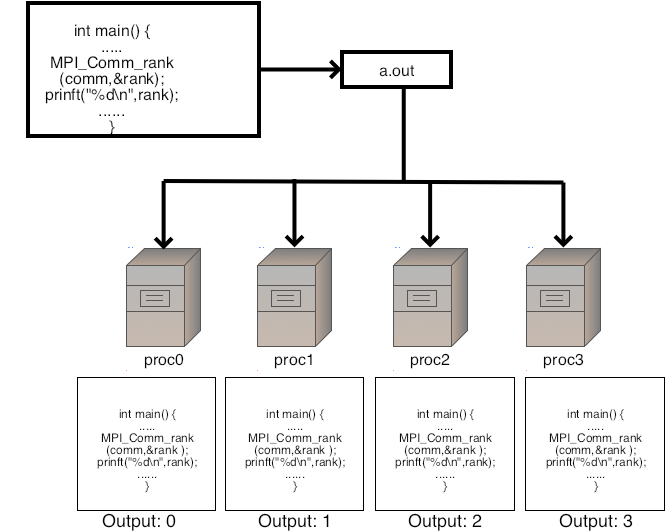FIGURE 2.5: Parallel program that prints process rank

In other words, each process can find out I am process 5 out of a total of 20'.

Exercise Write a program where each process prints out a message reporting its number, and how many processes there are:

Hello from process 2 out of 5!


Write a second version of this program, where each process opens a advisable if you have large numbers of processors, since it can overload the file system.} (There is a skeleton code commrank in the repository)
End of exercise

Exercise Write a program where only the process with number zero reports on how many processes there are in total.
End of exercise

In object-oriented approaches to MPI, that is, mpi4py and MPL  , the MPI_Comm_rank and MPI_Comm_size routines are methods of the communicator class:

Python note Rank and size are methods of the communicator object. Note that their names are slightly different from the MPI standard names.

comm = MPI.COMM_WORLD
procid = comm.Get_rank()
nprocs = comm.Get_size()


MPL note The rank of a process (by mpl::communicator:: rank) and the size of a communicator (by mpl::communicator:: size) are both methods of the communicator class:

const mpl::communicator &comm_world =
mpl::environment::comm_world();
int procid = comm_world.rank();
int nprocs = comm_world.size();

End of MPL note

## 2.4 Functional parallelism

crumb trail: > mpi-functional > Functional parallelism

Now that processes can distinguish themselves from each other, they can decide to engage in different activities. In an extreme case you could have a code that looks like

// climate simulation:
if (procid==0)
earth_model();
else if (procid=1)
sea_model();
else
air_model();

Practice is a little more complicated than this. But we will start exploring this notion of processes deciding on their activity based on their process number.

Being able to tell processes apart is already enough to write some applications, without knowing any other MPI. We will look at a simple parallel search algorithm: based on its rank, a processor can find its section of a search space. For instance, in Monte Carlo codes a large number of random samples is generated and some computation performed on each. (This particular example requires each MPI process to run an independent random number generator, which is not entirely trivial.)

Exercise Is the number $N=2,000,000,111$ prime? Let each process test a disjoint set of integers, and print out any factor they find. You don't have to test all integers $(Hint: i%0 probably gives a runtime error.) Can you find more than one solution? (There is a skeleton code prime in the repository) End of exercise Remark Normally, we expect parallel algorithms to be faster than sequential. Now consider the above exercise. Suppose the number we are testing is divisible by some small prime number, but every process has a large block of numbers to test. In that case the sequential algorithm would have been faster than the parallel one. Food for thought. End of remark As another example, in Boolean satisfiability problems a number of points in a search space needs to be evaluated. Knowing a process's rank is enough to let it generate its own portion of the search space. The computation of the Mandelbrot set can also be considered as a case of functional parallelism. However, the image that is constructed is data that needs to be kept on one processor, which breaks the symmetry of the parallel run. Of course, at the end of a functionally parallel run you need to summarize the results, for instance printing out some total. The mechanisms for that you will learn next. ## 2.5 Distributed computing and distributed data crumb trail: > mpi-functional > Distributed computing and distributed data One reason for using MPI is that sometimes you need to work on a single object, say a vector or a matrix, with a data size larger than can fit in the memory of a single processor. With distributed memory, each processor then gets a part of the whole data structure and only works on that. So let's say we have a large array, and we want to distribute the data over the processors. That means that, with p processes and n elements per processor, we have a total of$\mathtt{n}\cdot\mathtt{p}$elements.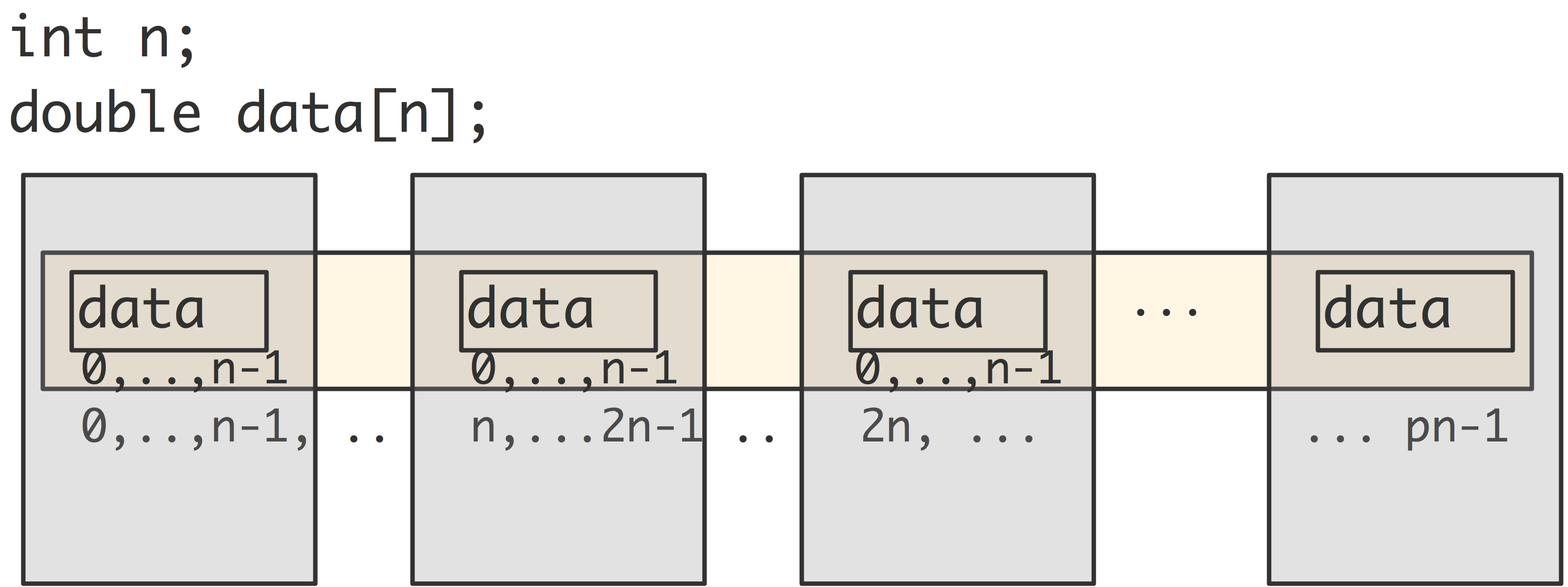FIGURE 2.6: Local parts of a distributed array We sometimes say that data is the local part of a distributed array with a total size of$\mathtt{n}\cdot\mathtt{p}$elements. However, this array only exists conceptually: each processor has an array with lowest index zero, and you have to translate that yourself to an index in the global array. In other words, you have to write your code in such a way that it acts like you're working with a large array that is distributed over the processors, while actually manipulating only the local arrays on the processors. Your typical code then looks like int myfirst = .....; for (int ilocal=0; ilocal<nlocal; ilocal++) { int iglobal = myfirst+ilocal; array[ilocal] = f(iglobal); }  Exercise Allocate on each process an array: int my_ints;  and fill it so that process 0 has the integers$0\cdots 9$, process 1 has$10\cdots 19\$, et cetera.

It may be hard to print the output in a non-messy way.
End of exercise

If the array size is not perfectly divisible by the number of processors, we have to come up with a division that is uneven, but not too much. You could for instance, write

int Nglobal, // is something large
Nlocal = Nglobal/ntids,
excess = Nglobal%ntids;
if (mytid==ntids-1)
Nlocal += excess;


Exercise Argue that this strategy is not optimal. Can you come up with a better distribution? Load balancing is further discussed in  Eijkhout:IntroHPC  .
End of exercise

## 2.6 Review questions

crumb trail: > mpi-functional > Review questions

For all true/false questions, if you answer that a statement is false, give a one-line explanation.

Exercise True or false: mpicc is a compiler.
End of exercise

Exercise T/F?

1. In C, the result of MPI_Comm_rank is a number from zero to number-of-processes-minus-one, inclusive.
2. In Fortran, the result of MPI_Comm_rank is a number from one to number-of-processes, inclusive.

End of exercise

Exercise What is the function of a hostfile?
End of exercise

\begin{pcse}

Exercise An architecture is called symmetric' or `uniform' if the relation between any pair of processes is essentially the same. In what way are MPI processes run on stampede symmetric; in what way not?
End of exercise

\end{pcse}